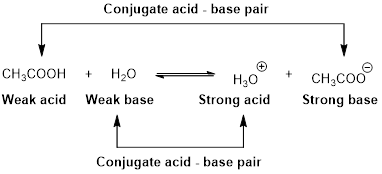Chapter 16.10, Problem 2.5ACP

Chapter
Section
Textbook Problem

To measure the relative strengths of bases stronger than OH−, it is necessary to choose a solvent that is a weaker acid than water. One such solvent is liquid ammonia. (a) Write a chemical equation for the autoionization of ammonia. (b) What is the strongest acid and base that can exist in liquid ammonia? (c) Will a solution of HCI in liquid ammonia be a strong electrical conductor, a weak conductor, or a nonconductor? (d) Oxide ion (O2−) is a stronger base than the amide ion (NH2−). Write an equation for the reaction of O2− with NH3 in liquid ammonia. Will the equilibrium favor products or reactants?

(a)

Interpretation Introduction

Interpretation:

The  chemical equation for the autoionization of ammonia has to be written.

Concept Introduction:

An equilibrium constant (K) is the ratio of the concentration of products and reactants raised to appropriate stoichiometric coefficient at equilibrium.

The reaction of an acid HA with water is written as,

HA(aq)+H2O(l)H3O+(aq)+A(aq)

The relative strength of an acid and base in water can be also expressed quantitatively with an equilibrium constant as follows:

Ka=[H3O+][A][HA]

An equilibrium constant (K) with subscript a indicates that it is an equilibrium constant of an acid in water.

The reaction of any base B with water is written as,

B(aq)+H2O(l)BH(aq)+OH(aq)

The relative strength of an acid and base in water can be also expressed quantitatively with an equilibrium constant as follows:

Kb=[BH][OH][B]

An equilibrium constant (K) with subscript b indicates that it is an equilibrium constant of the base in water.

Explanation

Ammonia is similar to water that it can both act as an acid and a base. It is a weak acid or base that it can not completely dissociate in a solution.

The chemical equation for the autoionization of liquid ammonia is written as,

2NH

(b)

Interpretation Introduction

Interpretation:

The Strongest acid and base that can exist in liquid ammonia has to be identified.

Concept Introduction:

Arrhenius Acids and Bases:

Acid release hydrogen ion in water

Base release hydroxide ions in water.

HCl(aq) H+(aq) + Cl-(aq).........AcidNaOH(aq) Na+(aq)+ OH-(aq)...........Base

An acid is a substance that produces hydronium ions, H3O+ when dissolved in water.

Bronsted –Lowry definitions:

A Bronsted –Lowry acid is a proton donor, it donates a hydrogen ion, H+

A Bronsted-Lowry base is a proton acceptor, it accepts a hydrogen ion H+Lewis definition:

A Lewis acid is a substance that can accept and share an electron pair.

A Lewis base is a substance that can donate and share an electron pair.Bronsted –Lowry conjugate acid-Base pairs:

When an acid is dissolved in water, the acid (HA) donates a proton to water to form a new acid (conjugate acid) and a new base (conjugate base).The pair of an Acid –Base differs by a proton called conjugated Acid-Base pair.

(c)

Interpretation Introduction

Interpretation:

A solution of HCl in liquid ammonia be a good conductor, bad conductor or nonconductor of electricity has to be explained.

Concept Introduction:

Conductor: It is a type of material and it allows the flow of an electrical current in one or more directions.

An acid when dissolved in a solution, it has the following properties;

It is a good conductor of electricity.

It changes the color of litmus paper from blue to red.

It tastes sour.

It reacts with a base to produce salt and water.

Non-conductor: It does not conduct the electricity.

(d)

Interpretation Introduction

Interpretation:

The equation for the reaction of O2 with NH3 has to be written and the reaction is a reactant or product favored at equilibrium is to be stated.

Concept Introduction:

An equilibrium constant (K) is the ratio of the concentration of products and reactants raised to appropriate stoichiometric coefficient at equilibrium.

The reaction of an acid HA with water is written as,

HA(aq)+H2O(l)H3O+(aq)+A(aq)

The relative strength of an acid and base in water can be also expressed quantitatively with an equilibrium constant as follows:

Ka=[H3O+][A][HA]

An equilibrium constant (K) with subscript a indicates that it is an equilibrium constant of an acid in water.

The reaction of any base B with water is written as,

B(aq)+H2O(l)BH(aq)+OH(aq)

The relative strength of an acid and base in water can be also expressed quantitatively with an equilibrium constant as follows:

Kb=[BH][OH][B]

An equilibrium constant (K) with subscript b indicates that it is an equilibrium constant of the base in water.

Still sussing out bartleby?

Check out a sample textbook solution.

See a sample solution

The Solution to Your Study Problems

Bartleby provides explanations to thousands of textbook problems written by our experts, many with advanced degrees!

Get Started